## Calculated Extinction Curve

The three main contributions to extinction by the Earth's atmosphere of relevance to ground-based astronomy are Rayleigh scattering by air molecules, molecular absorption and aerosol scattering. The method outlined in Hayes and Latham (1975) gives the mean extinction for an aerosol-free atmosphere, as follows:

1. Rayleigh scattering. The wavelength (inm) and altitude (h in km) dependence of Rayleigh vertical extinction is approximated by: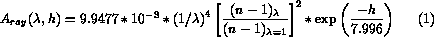where the scale height of the lower troposphere is taken to be 7.996 km and h = 2.369 km for the Roque de los Muchachos Observatory.

The index of refraction term is given by: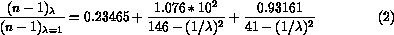2. Molecular absorption. The major contributors are absorption bands of ozone and water vapour. The latter is not included in the calculated extinction curve, because the amount of water vapour above any observatory is extremely variable. Water vapour bands can contribute more than 0.01 mag extinction per unit air mass at 7100, 8090, 9700 and 10800Å.

Vertical extinction by ozone is approximated by: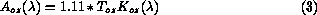where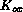(cm) is the absorption coefficient (Gast 1960) and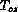(atm cm) is the total ozone above the observatory.is independent of the observatory altitude, since atmospheric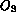is concentrated between 10 and 35 km. It does, however, exhibit seasonal variations and it can also vary significantly on time scales as short as a few hours. The extinction curve here uses a mean annual value ofappropriate to the latitude of La Palma, taken from Allen (1963).

The total vertical extinction coefficient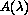for an aerosol-free atmosphere is then given by;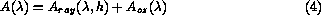Values ofhave been calculated every 10Å between 3000 and 3500Å and every 50Å between 3500 and 11000Å and are collected in Table 1. The extinction curve is shown in Figure 1, together with preliminiary measurements obtained on dust-free nights (Andrews 1985, private communication).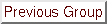Previous: Introduction
Up: Extinction
Next: Correction for Dust Extinction
Previous Page: Introduction
Next Page: Correction for Dust Extinction

Fri Jun 10 17:31:56 BST 1994